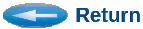## I-GUI Example

This example is a very simple (and pointless) one-box system model that contains a global attribute (in this case, a macro), called X. The model does nothing but write the result.dat file, each time it is run. It writes one line into the file. The line contains two values, X, and X squared.

The suggested example is to use the ITERATOR to sweep the X value from 1 to 10, by steps of one. And to plot the second column as a function of the first column. This should reveal the familiar Y equals X squared curve. A second aspect is to print the statistics of the second column (X^2), and a third would be to plot it's histogram. Suggested histogram parameters would be: range=0:100, #bins=5.

Detailed Instructions:

```1. Download "test_iterator.sim", and build the simulation:
Either:
gui test_iterator.sim
(then click Tools/Build)
Or just type:
csim test_iterator.sim

2. Open the Iterator GUI.
(click Tools / Iterator)
Or just type:
igui

Once in the Iterator GUI, do the following:
A. Click "Control Variables"
Click "Sweep Parameter"
Fill in the fields:
Attribute Name = X
Initial Value = 1
Final Value = 10
Step Size = 1
Click OK.
Dismiss Control Variable window.
B. Click "Run Controls"
Defaults should be good.
Click "Ok".
C. Click "Results Collection/Plots"
Click "Plot Values"
Fill in the fields:
X column number: 1
Y column number: 2
(Explanation:  If you look at the simple model, it writes two numbers to
the results.dat file each run.  These become two columns of numbers.
The first column is the "X" value.  The second column is the "X^2" value.
We want to plot "X vs. X^2", which is column 1 vs. column 2.
Column 1 becomes the X-axis value on the plot, and column 2 becomes
the Y-axis value on the standard (x vs. y) plot.
)
Red
(Other fields are optional.)
Click "Ok".
Dismiss Results Collection window.
D. Click "Start Iterator - Run Simulations"
It will ask you to save your selections.  Save to default file.
You can review your iterator-control file if you wish.
Then start the runs.
You should see the simulation run ten times.
Now you are ready to view the results.
E. Click "List/Plot Results".
Click "Plot Grpahs"
You should see a list of your plots, which should contain your one plot.
Select your plot, and click "Plot It".